# AP Calculus AB Practice Test 48

### Test Information10 questions20 minutes

Calculator Disallowed

1. The area of the region bounded by the curves of y= -x2and y= xis

2.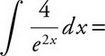3. If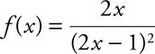, then f′(x)=

4. Let fand gbe differentiable functions such that f-1(x) = g(x) for all x. The table below shows selected values of f(x) and f′(x). What is the value of g′(2)?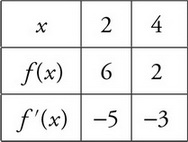5.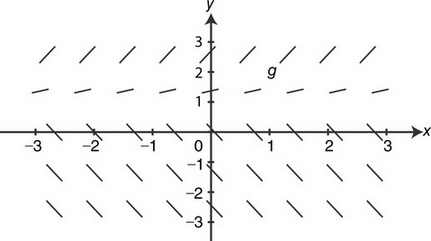The figure above shows the slope field for which of the following differential equations?

6. Let fbe a continuous and twice differentiable function and f″(x) 0 for all xin the interval [4, 5]. Some of the values of fare shown in the table below.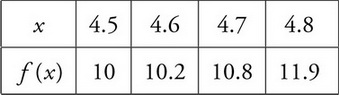Which of the following is true about f′(4.6)?

7.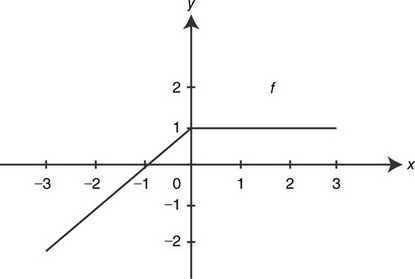The graph of a function fis shown in the figure above. The graph of fconsists of two line segments, and fis continuous on the interval [-3, 3]. If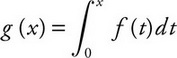, then g(-3) is

8. The solution to the differential equation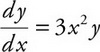where y(0) = eis

9. The graph of a continuous function y= f(x) passes through the point (e, 4) and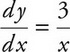. Which of the following is the function f(x)?

10. The definite integral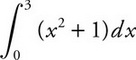is equivalent to which of the following limits?